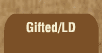Mathematics and Dyslexia

Tips for Learning the Times Tables

Tools for the Times Tables & Workbook

Home > Math > times tables practice > Eights (low, scrambled)

## The Eight Times Tables - first set

Technically, you should know these already -- they've all been covered under the other tables. Make sure they're not rusty, though! If you have trouble, go back and try them in order. You may notice that to get to the next "eights" table, you can add ten then back up two... so you'd think "hmmm... 8 x 3 is 24... add ten for 34... back up to 32... so 8 x 4 is 32."

1. 8 x 3=
2. 3 x 8=
3. 8 x 5=
4. 1 x 8=
5. 8 x 0=
6. 2 x 8=
7. 8 x 4=
8. 8 x 3=
9. 5 x 8=
10. 1 x 8=
11. 8 x 2=
12. 4 x 8=
13. 3 x 8=
14. 8 x 4=
15. 5 x 8=